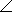# Electrical Engineering - RC Circuits

### Exercise :: RC Circuits - General Questions

26.

A capacitor with 150of capacitive reactance is across an ac source. The impedance, expressed in polar form, is

 A. Z = 150 B. Z = 15090°C. Z = 150–90°D. Z = 150180°Answer: Option C

Explanation:

No answer description available for this question. Let us discuss.

27.

When the frequency of the voltage applied to a series RC circuit is decreased, the impedance

 A. increases B. decreases C. remains the same D. doubles

Answer: Option A

Explanation:

No answer description available for this question. Let us discuss.

#### Current Affairs 2021

Interview Questions and Answers